# Math in Focus Grade 5 Chapter 4 Practice 5 Answer Key Real-World Problems: Multiplying Mixed Numbers

Go through the Math in Focus Grade 5 Workbook Answer Key Chapter 4 Practice 5 Real-World Problems: Multiplying Mixed Numbers to finish your assignments.

## Math in Focus Grade 5 Chapter 4 Practice 5 Answer Key Real-World Problems: Multiplying Mixed Numbers

Question 1.
At a party, there are 8 guests. Each guest eats 2$$\frac{1}{4}$$ oranges.
How many oranges do the 8 guests eat?
1 guest → 2$$\frac{1}{4}$$ oranges
8 guests → ___ × ____ oranges
= _____
The 8 guests eat a total of ___ oranges.
The 8 guests eat a total of 18
oranges.
Explanation:
1 guest → 2$$\frac{1}{4}$$ oranges
8 guests → 8 × $$\frac{9}{4}$$  oranges
= 18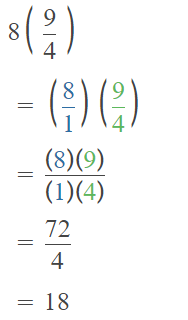Question 2.
One pound of chicken costs $3. Jim buys 8$$\frac{2}{3}$$ pounds of chicken. How much does Jim pay for the chicken? Answer: One pound of chicken =$3
8$$\frac{2}{3}$$ x 3
Explanation: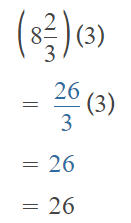Question 3.
Nolan practices the piano for 1$$\frac{2}{5}$$ hours every Saturday and Sunday. How long does he practice each weekend? Express your answer in hours and minutes.
he practiced 2 hours 48 minutes
Explanation:
1$$\frac{2}{5}$$ hours on Saturday
1$$\frac{2}{5}$$ hours on Sunday
1$$\frac{2}{5}$$ + 1$$\frac{2}{5}$$
2$$\frac{4}{5}$$
1 hour = 60 minutes
5 parts of 60 = 5 x 12 = 60
60 – 12 = 48
so, he practiced 2 hours 48 minutes

Question 4.
Sue buys 5 pieces of fabric. Each piece of fabric is 1$$\frac{7}{10}$$ yards long.
a. What is the total length of the fabric she buys?
Sue buys 5 pieces of fabric.
Each piece of fabric is 1$$\frac{7}{10}$$ yards long.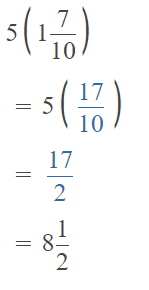b. One yard of the fabric costs $5. How much does she pay for all 5 pieces of fabric? Answer: One yard of the fabric costs$5.
she pay for all 5 pieces of fabric
5 x 5 = 25
She paid $25 Question 5. Angela works 1$$\frac{1}{2}$$ hours a day and is paid$7 per hour. She works 5 days a week. How much does Angela earn in 7 weeks?
Angela works 1$$\frac{1}{2}$$ hours a day and is paid \$7 per hour.
1$$\frac{1}{2}$$ x 7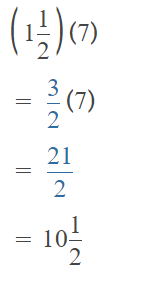She works 5 days a week.
5 x 10$$\frac{1}{2}$$
= 50$$\frac{5}{2}$$
= 52$$\frac{1}{2}$$
Angela earn in 7 weeks
7 x 52$$\frac{1}{2}$$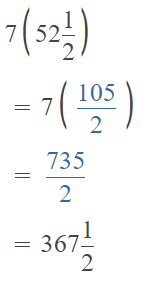Explanation:
7 x 5 = 35
35 x 7 = 245
245 x 1$$\frac{1}{2}$$
367$$\frac{1}{2}$$